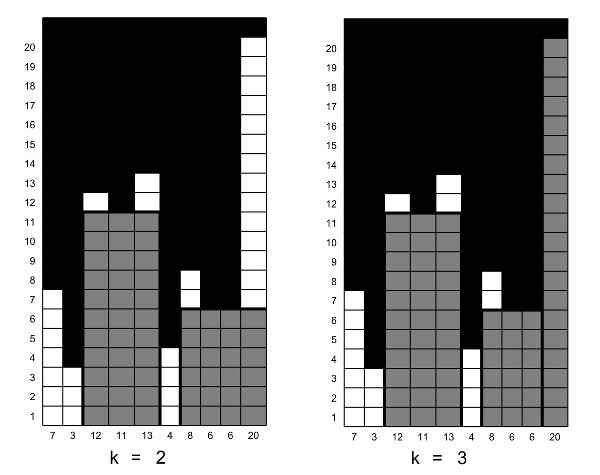STREET - Street

There are n lots on one side of a street (where n ≤ 500). We would like to erect at most k apartment buildings on these lots. Each building must occupy an interval of at most t consecutive lots. Moreover, each lot i has a height restriction r[i] (where r[i] ≤ 100). A building cannot exceed any of the height restriction of any lot on which it is built (that is, the maximal height of the building that can be erected on lot i to j is:

H = min{r[i], r[i + 1], ..., r[j]})

Hence, the maximum usable facade space of the building is: H × (j − i + 1). We would like to have a program to select at most k non-overlapping intervals to erect the buildings such that the total usable facade space is maximized.

Example 1

Consider a street of length 10. The height restriction of each lot is as follows:

7, 3, 12, 11, 13, 4, 8, 6, 6, 20

Suppose we would like to erect at most k = 2 buildings and each building occu- pies at most t = 4 lots. Then, to maximize the total usable facade space, we choose two intervals r[3..5] = (12, 11, 13) and r[7..10] = (8, 6, 6, 20) (see “Example 1” in the figure below). The maximum usable facade space is 3 ∗ min{12, 11, 13} + 4 ∗ min{8, 6, 6, 20} = 57.Example 2

Suppose we would like to erect at most k = 3 buildings on the same street with the same height restrictions as in Example 1, and each building occupies at most t = 4 lots. Then, to maximize the total usable facade space, we choose three intervals r[3..5] = (12, 11, 13), r[7..9] = (8, 6, 6) and r[10..10] = (20) (see “Example 2” in the figure above). The maximum usable facade space is 3 ∗ min{12, 11, 13} + 3 ∗ min{8, 6, 6} + 1 ∗ 20 = 71.

Input

The input file is as follows: The first line contains three integers n, k, and t separated by a space character, where 1 ≤ n ≤ 500, 1 ≤ k ≤ n, and 1 ≤ t ≤ n. The rest of the nlines contain n positive integers representing the height restriction for the n lots. For Example 1, the input file looks like:

10 2 4
7
3
12
11
13
4
8
6
6
20

The input should be read from the standard input, and your program will be run several times, each one with one of the test cases.

Output

The output file contains an integer which is the maximum usable facade space. For the above example, the output file looks like:

57

National Olympiad in Informatics (NOI) - 2007altuner: 2019-02-27 19:47:41 hardester Last edit: 2019-02-27 19:47:52vudet22: 2018-06-21 05:49:27 Useless t lmao.k_nushka: 2017-07-10 14:08:19 Easy. :)mathador: 2016-08-15 21:01:47 easy peasy ;)mmz33: 2016-08-15 21:00:44 easy peasy! ;)Piyush Kumar: 2016-06-19 04:33:29 The picture is not visible! =(Francky)=> mail send to admin ; I failed for this case. Last edit: 2016-06-19 12:43:15kejriwal: 2015-11-16 13:05:56 nice one :D !!s1306: 2015-09-25 08:58:30 state is critical,do not include "t" in your state,include "i" in-dices(from left) and "j" buildings erected on them.rahul kumar singh: 2015-08-07 17:50:31 easy one